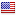#### Join the players club!

I see you dont have an account, join the club to get acces to everything! Make friends, keep track of scores, discover courses and more.## High Bridge Hills Golf Club

Par:
71
Total distance:
2019 Meters
Country:City:
High Bridge, NJ
golf_course Hole 1 2 3 4 5 6 7 8 9 10 11 12 13 14 15 16 17 18 Total
Par 5 3 5 3 4 3 5 3 4 4 3 4 3 4 4 4 5 5 71
Distance 233m 66m 165m 57m 114m 69m 152m 58m 105m 100m 74m 92m 48m 117m 107m 106m 206m 153m 2019m
Strokeindex 0 0 0 0 0 0 0 0 0 0 0 0 0 0 0 0 0 0 -

### Last 10 rounds

• Date
Course
Description
• ```stdClass Object
(
[_id] => 596e302af509273285318045
[by] => stdClass Object
(
)

[coursename] => High Bridge Hills Golf Club
[course] => stdClass Object
(
[_id] => 5966df18f5092759106d2753
[name] => High Bridge Hills Golf Club
[footgolfclub] =>
[country] => US
[city] => High Bridge, NJ
[total] => stdClass Object
(
[par] => 71
[meters] => 2019.026
)

[holes] => Array
(
 => stdClass Object
(
[par] => 5
[meters] => 233.07
[strokeindex] => 0
)

 => stdClass Object
(
[par] => 3
[meters] => 65.808
[strokeindex] => 0
)

 => stdClass Object
(
[par] => 5
[meters] => 164.52
[strokeindex] => 0
)

 => stdClass Object
(
[par] => 3
[meters] => 56.668
[strokeindex] => 0
)

 => stdClass Object
(
[par] => 4
[meters] => 114.25
[strokeindex] => 0
)

 => stdClass Object
(
[par] => 3
[meters] => 68.55
[strokeindex] => 0
)

 => stdClass Object
(
[par] => 5
[meters] => 151.724
[strokeindex] => 0
)

 => stdClass Object
(
[par] => 3
[meters] => 57.582
[strokeindex] => 0
)

 => stdClass Object
(
[par] => 4
[meters] => 105.11
[strokeindex] => 0
)

 => stdClass Object
(
[par] => 4
[meters] => 99.626
[strokeindex] => 0
)

 => stdClass Object
(
[par] => 3
[meters] => 74.034
[strokeindex] => 0
)

 => stdClass Object
(
[par] => 4
[meters] => 92.314
[strokeindex] => 0
)

 => stdClass Object
(
[par] => 3
[meters] => 47.528
[strokeindex] => 0
)

 => stdClass Object
(
[par] => 4
[meters] => 116.992
[strokeindex] => 0
)

 => stdClass Object
(
[par] => 4
[meters] => 106.938
[strokeindex] => 0
)

 => stdClass Object
(
[par] => 4
[meters] => 106.024
[strokeindex] => 0
)

 => stdClass Object
(
[par] => 5
[meters] => 205.65
[strokeindex] => 0
)

 => stdClass Object
(
[par] => 5
[meters] => 152.638
[strokeindex] => 0
)

)

[numholes] => 18
[first9] => stdClass Object
(
[par] => 35
[meters] => 1017.282
)

[second9] => stdClass Object
(
[par] => 36
[meters] => 1001.744
)

[logo] => true
[photo] => true
[player] => stdClass Object
(
)

[metrics] => yards
[active] => true
[email] => true
[followers] => Array
(
)

)

[description] => USGA FOX CREW
[roundtype] => fun
[flight] => Array
(
 => stdClass Object
(
[personName] => Kevin Norris
[photo] =>
[holes] => Array
(
 => stdClass Object
(
[round] =>
[course] => 5966df18f5092759106d2753
[hole] => 0
[holepar] => 5
[scoretrack] => 0
[score] => 5
)

 => stdClass Object
(
[round] =>
[course] => 5966df18f5092759106d2753
[hole] => 1
[holepar] => 3
[scoretrack] => 0
[score] => 3
)

 => stdClass Object
(
[round] =>
[course] => 5966df18f5092759106d2753
[hole] => 2
[holepar] => 5
[scoretrack] => -1
[score] => 4
)

 => stdClass Object
(
[round] =>
[course] => 5966df18f5092759106d2753
[hole] => 3
[holepar] => 3
[scoretrack] => 0
[score] => 3
)

 => stdClass Object
(
[round] =>
[course] => 5966df18f5092759106d2753
[hole] => 4
[holepar] => 4
[scoretrack] => -1
[score] => 3
)

 => stdClass Object
(
[round] =>
[course] => 5966df18f5092759106d2753
[hole] => 5
[holepar] => 3
[scoretrack] => 0
[score] => 3
)

 => stdClass Object
(
[round] =>
[course] => 5966df18f5092759106d2753
[hole] => 6
[holepar] => 5
[scoretrack] => -1
[score] => 4
)

 => stdClass Object
(
[round] =>
[course] => 5966df18f5092759106d2753
[hole] => 7
[holepar] => 3
[scoretrack] => -1
[score] => 2
)

 => stdClass Object
(
[round] =>
[course] => 5966df18f5092759106d2753
[hole] => 8
[holepar] => 4
[scoretrack] => 1
[score] => 5
)

 => stdClass Object
(
[round] =>
[course] => 5966df18f5092759106d2753
[hole] => 9
[holepar] => 4
[scoretrack] => -1
[score] => 3
)

 => stdClass Object
(
[round] =>
[course] => 5966df18f5092759106d2753
[hole] => 10
[holepar] => 3
[scoretrack] => 0
[score] => 3
)

 => stdClass Object
(
[round] =>
[course] => 5966df18f5092759106d2753
[hole] => 11
[holepar] => 4
[scoretrack] => 0
[score] => 4
)

 => stdClass Object
(
[round] =>
[course] => 5966df18f5092759106d2753
[hole] => 12
[holepar] => 3
[scoretrack] => 0
[score] => 3
)

 => stdClass Object
(
[round] =>
[course] => 5966df18f5092759106d2753
[hole] => 13
[holepar] => 4
[scoretrack] => 0
[score] => 4
)

 => stdClass Object
(
[round] =>
[course] => 5966df18f5092759106d2753
[hole] => 14
[holepar] => 4
[scoretrack] => -1
[score] => 3
)

 => stdClass Object
(
[round] =>
[course] => 5966df18f5092759106d2753
[hole] => 15
[holepar] => 4
[scoretrack] => -1
[score] => 3
)

 => stdClass Object
(
[round] =>
[course] => 5966df18f5092759106d2753
[hole] => 16
[holepar] => 5
[scoretrack] => 0
[score] => 5
)

 => stdClass Object
(
[round] =>
[course] => 5966df18f5092759106d2753
[hole] => 17
[holepar] => 5
[scoretrack] => 0
[score] => 5
)

)

[country] => US
[totals] => stdClass Object
(
[totalScore] => -6
[totalShots] => 65
)

)

)

[open] =>
[startdate] => stdClass Object
(
[\$date] => 1500394500000
[\$unixtime] => 1500394500
[_datetime] => 2017-07-18 18:15:00
)

[numholes18] =>
[likes] => Array
(
)

)
```